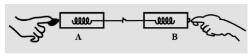Courses

# Test: Newton Third Law Of Motion

## 10 Questions MCQ Test Science Class 9 | Test: Newton Third Law Of Motion

Description
This mock test of Test: Newton Third Law Of Motion for Class 9 helps you for every Class 9 entrance exam. This contains 10 Multiple Choice Questions for Class 9 Test: Newton Third Law Of Motion (mcq) to study with solutions a complete question bank. The solved questions answers in this Test: Newton Third Law Of Motion quiz give you a good mix of easy questions and tough questions. Class 9 students definitely take this Test: Newton Third Law Of Motion exercise for a better result in the exam. You can find other Test: Newton Third Law Of Motion extra questions, long questions & short questions for Class 9 on EduRev as well by searching above.
QUESTION: 1

### A child on a cart with wheels throws a sandbag forward. As a result:

Solution:

The child moves in the backward direction as a consequence of action-reaction law. Sandbag exerts an equal and opposite force in the backward direction.

QUESTION: 2

### When a fireman directs a powerful stream of water from a hose-pipe, the hose-pipe tends to go backward. This is due to:

Solution:
• According to the circumstances mentioned in the question, a powerful stream of water on a fire from a hose-pipe tend to go backward.
• This phenomenon is the practical life example of Newton's third law, " Every action has an equal and opposite reaction."
QUESTION: 3

### Which among the following is true as per Newton’s third law:

Solution:
QUESTION: 4

Action and reaction forces are always:

Solution:
• It is Newton's third law of motion which states that every action has an equal and opposite reaction.
• So, every action and reaction forces are equal and in opposite direction.
QUESTION: 5

A person standing on a weighing scale sees a reading on the scale as 148 pounds. This person is acted on by:

Solution:
• Gravitational force is acting in the downward direction. As we know every action has a reaction.
• So, there is an equal and opposite force acting in opposite direction also.
QUESTION: 6

An archer shoots an arrow. Consider the action force to be the bow string against the arrow. The reaction force is the:

Solution:

According to Newton's 3rd law of motion, " Every action has an equal and opposite reaction ".  So if we have to push the arrow in forward direction, we will pull the bow string in backward direction.

QUESTION: 7

Two spring balances pull each other in opposite directions. If the reading of one spring balance is 2 N, the reading shown by the second spring balance will be:

Solution:The spring balances are hooked and pulled in opposite direction. By the action-reaction pair force, the reading must be equal to 2.0 N.

QUESTION: 8

Which one is correct if a bullet is fired from a rifle?

Solution:
• When a bullet is fired from a rifle, the rifle exerts a force on the bullet in the forward direction. This is the action force.
• The bullet also exerts an equal force on the rifle in the backward direction. This is the reaction force.
• So because of this action-reaction pair force, the bullet moves in the forward direction and the rifle moves in the backward direction.
QUESTION: 9

Which is incorrect statement about action and reaction forces?

Solution:
• The action and reaction force always act on different objects.
• Two forces acting on the same object, even if they have the same magnitude and point in the opposite direction, never form an action-reaction pair.
QUESTION: 10

A player is catching a ball. Consider the action force to be the impact of the ball against the player’s glove. What is the reaction force?

Solution:

Reaction force: Force that the glove exerts on the ball is the reaction force, opposite to the impact of the ball on the player’s glove.